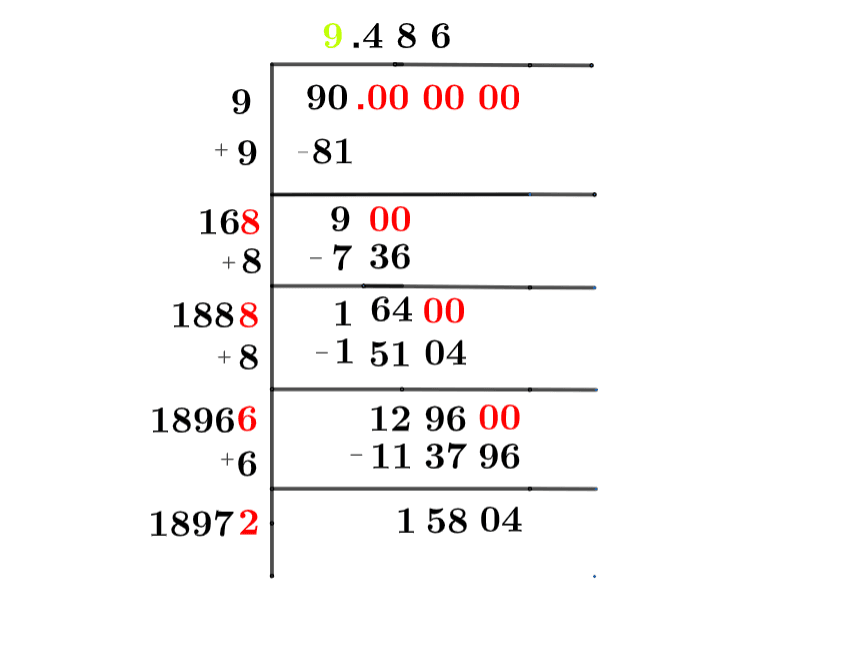Contents

Square Root of 90 + Solution With Free Steps

In this solution, we will see how we can calculate the square root of the number 90 which is less than 100 which is written in terms of the square root symbol as √90, it may be a perfect square number or maybe not a perfect square number.

In this article, we will analyze and find the square root of 90 using various mathematical techniques, such as the approximation method and the long division method.

## What Is the Square Root Of 90?

The square root of the number 90 is 9.486.

The square root can be defined as the quantity that can be doubled to produce the square of that similar quantity. In simple words, it can be explained as:

√90 = √(9.486 x 9.486)

√90 = √(9.486)$^2$

√90 = ±9.486

The square can be canceled with the square root as it is equivalent to 1/2; therefore, obtaining 9.486. Hence 9.486 is 90’s square root. The square root generates both positive and negative integers.

## How To Calculate the Square Root of 90?

You can calculate the square root of 90 using any of two vastly used techniques in mathematics; one is the Approximation technique, and the other is the Long Division method.

The symbol √ is interpreted as 90 raised to the power 1/2. So any number, when multiplied by itself, produces its square, and when the square root of any squared number is taken, it produces the actual number.

Let us discuss each of them to understand the concepts better.

### Square Root by Long Division Method

The process of long division is one of the most common methods used to find the square roots of a given number. It is easy to comprehend and provides more reliable and accurate answers. The long division method reduces a multi-digit number to its equal parts.

Learning how to find the square root of a number is easy with the long division method. All you need are five primary operations- divide, multiply, subtract, bring down or raise, then repeat.

Following are the simple steps that must be followed to find the square root of 90 using the long division method:

### Step 1

First, write the given number 90 in the division symbol, as shown in figure 1.

### Step 2

Starting from the right side of the number, divide the number 90 into pairs such as 00 and 90.

### Step 3

Now divide the digit 90 by a number, giving a number either 90 or less than 90. Therefore, in this case, the remainder is 9, whereas the quotient is 9.

### Step 4

After this, bring down the next pair 00. Now the dividend is 900. To find the next divisor, we need to double our quotient obtained before. Doubling 9 gives 18; hence consider it as the next divisor.

### Step 5

Now pair 16 with another number to make a new divisor that results in $\leq$ 900 when multiplied with the divisor. If the number is not a perfect square, add pair of zeros to the right of the number before starting division.

### Step 6

Adding 8 to the divisor and multiplying 168 with 8 results in 736 $\leq$ 900. The remainder obtained is 164. Move the next pair of zeros down and repeat the same process mentioned above.

### Step 7

Keep on repeating the same steps till the zero remainder is obtained or if the division process continues infinitely, solve to two decimal places.

### Step 8

The resulting quotient 9.486 is the square root of 90. Figure 1 given below shows the long division process in detail:figure 1

### Square Root by Approximation Method

The approximation method involves guessing the square root of the non-perfect square number by dividing it by the perfect square lesser or greater than that number and taking the average.

The given detailed steps must be followed to find the square root of 90 using the approximation technique.

### Step 1

Consider a perfect square number 81 less than 90.

### Step 2

Now divide 90 by 9.

90 ÷ 9 = 10

### Step 3

Now take the average of 9 and 10. The resulting number is approximately equivalent to the square root of 90.

(9 + 10) ÷ 2 = 9.5

### Important points

• The number 90 is not a perfect square.
• The number 90 is a rational number.
• The number 90 can be split into its prime factorization.

## Is Square Root of 90 a Perfect Square?

The number 90 is not a perfect square. A number is a perfect square if it splits into two equal parts or identical whole numbers. If a number is a perfect square, it is also rational.

A number expressed in p/q form is called a rational number. All the natural numbers are rational. A square root of a perfect square is a whole number; therefore, a perfect square is a rational number.

A number that is not a perfect square is irrational as it is a decimal number. As far as 90 is concerned, it is not a perfect square. It can be proved as below:

Factorization of 90 results in 2 x 45.

Taking the square root of the above expression gives:

= √(2 x 45)

= (2 x 45)$^{1/2}$

= 9.486

This shows that 90 is not a perfect square as it has decimal places; hence it is an irrational number.

Therefore the above discussion proves that the square root of 90 is equivalent to 9.486.

Images/mathematical drawings are created with GeoGebra.

You are watching: Square Root of 90+ Solution With Free Steps. Info created by GBee English Center selection and synthesis along with other related topics.